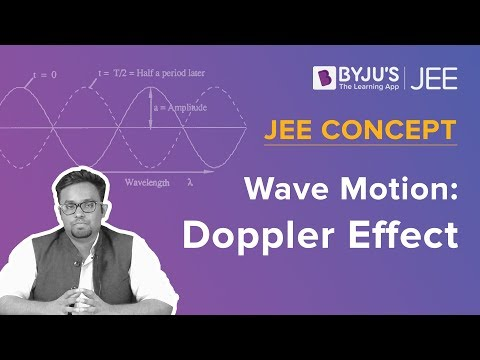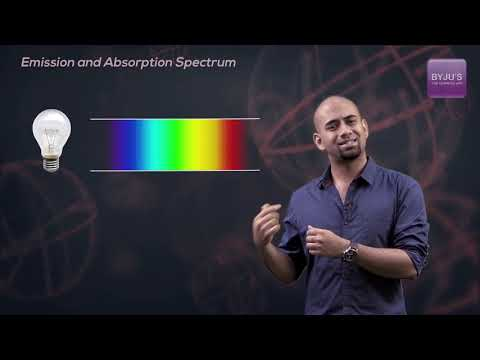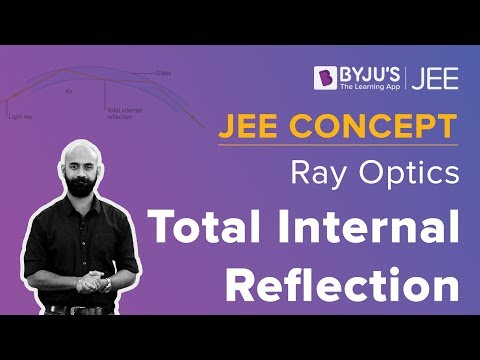Win up to 100% scholarship on Aakash BYJU'S JEE/NEET courses with ABNAT Win up to 100% scholarship on Aakash BYJU'S JEE/NEET courses with ABNAT

# JEE Main 2019 Physics Paper With Solutions January 11 Shift 1

Students can find the JEE Main 2019 (Shift 1-January 11) Physics question paper with solutions on this page. All these solutions are solved by the subject experts at BYJU’S. Solving previous years’ question papers help the students in understanding the coverage of the topics as well as the pattern of the paper. These questions are answered in the simplest possible method.
January 11 – Physics Shift 1

1. The force of interaction between two atoms is given by

$$\begin{array}{l}F = \alpha \beta exp\left [ \frac{-x^{2}}{\alpha kt} \right ]\end{array}$$
; where x is the distance, k is the Boltzmann constant and T is temperature
$$\begin{array}{l}\alpha\end{array}$$
and
$$\begin{array}{l}\beta\end{array}$$
are two constants. The dimension of
$$\begin{array}{l}\beta\end{array}$$
is:

a. M2L2T–2
b. M2LT–4
c. M0L2T–4
d. MLT–2

$$\begin{array}{l}F=\alpha \beta e^{\frac{-x^{2}}{\alpha KT}}\end{array}$$
$$\begin{array}{l}\left [ \frac{x^{2}}{\alpha KT} \right ]=M^{0}L^{0}T^{0}\end{array}$$
$$\begin{array}{l}\left [ \frac{L^{2}}{\alpha ML^{2}T^{-2}} \right ]=M^{0}L^{0}T^{0}\end{array}$$
$$\begin{array}{l}\left [ \alpha \right ]=M^{-1}T^{2}\end{array}$$
$$\begin{array}{l}\left [ F \right ]= \left [ \alpha \right ]\left [ \beta \right ]\end{array}$$
$$\begin{array}{l}\left [ MLT^{-2} \right ]= M^{-1}T^{2}\left [ \beta \right ]\end{array}$$
$$\begin{array}{l}\left [ \beta \right ]= M^{2}LT^{-4}\end{array}$$

2. A particle is moving along a circular path with a constant speed of 10 ms–1. What is the magnitude of the change in velocity of the particle, when it moves through an angle of 60° around the centre of the circle?

a. zero
b. 10 m/s
c.

$$\begin{array}{l}10\sqrt{3}\end{array}$$
m/s

d.
$$\begin{array}{l}10\sqrt{2}\end{array}$$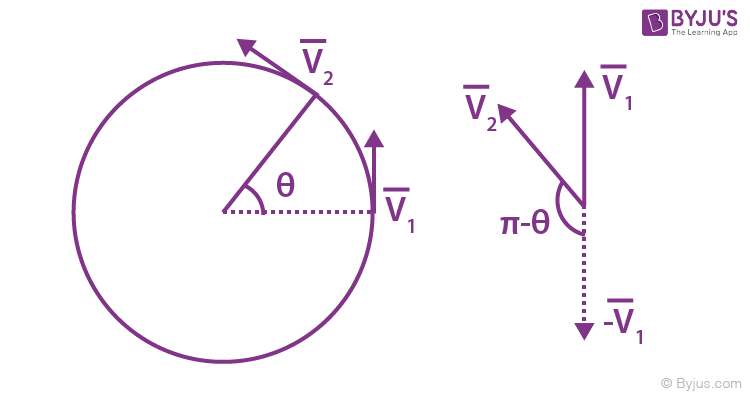$$\begin{array}{l}\left | \Delta \vec{v} \right |=\sqrt{v_{1}^{2}+v_{2}^{2}+2v_{1}v_{2}cos(\pi -\theta )}\end{array}$$

=

$$\begin{array}{l}2vsin\frac{\theta }{2}\end{array}$$
(since
$$\begin{array}{l}\left | \bar{v_{1}} \right |=\left | \bar{v_{2}} \right |\end{array}$$

= (2 x 10) x sin(300)

= 10 m/s

3. A body is projected at t = 0 with a velocity 10 ms–1 at an angle of 60° with the horizontal.

The radius of curvature of its trajectory at t = 1s is R. Neglecting air resistance and takin acceleration due to gravity g = 10 ms–2, the value of R is :

a. 2.5 m
b. 10.3 m
c. 2.8 m
d. 5.1 m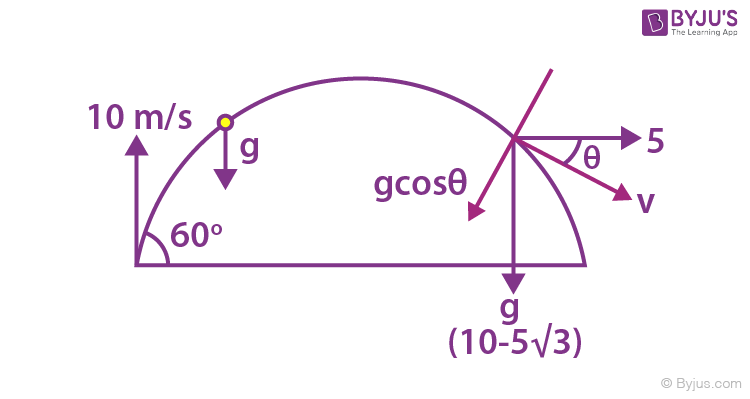vx = 10cos60° = 5 m/s

vy = 10cos30° =

$$\begin{array}{l}5\sqrt{3}\end{array}$$
m/s

velocity after t = 1 sec.

$$\begin{array}{l}v_{y}=\left | (5\sqrt{3}-10) \right |=10-5\sqrt{3}\end{array}$$
$$\begin{array}{l}a_{n}=\frac{v^{2}}{R}=\frac{v_{x}^{2}+v_{y}^{2}}{a_{n}}\end{array}$$

$$\begin{array}{l}a_{n}=\frac{25+100+75-100\sqrt{3}}{10cos\theta }\end{array}$$
$$\begin{array}{l}tan \theta =\frac{10-5\sqrt{3}}{5}=2-\sqrt{3}\Rightarrow \theta =15^{0}\end{array}$$
$$\begin{array}{l}R = \frac{100(2-\sqrt{3})}{10cos15}=2.8 m\end{array}$$

4. A liquid of density

$$\begin{array}{l}\rho\end{array}$$
is coming out of a hose pipe of radius a with horizontal speed v and hits a mesh. 50% of the liquid passes through the mesh unaffected. 25% looses all of its momentum and 25% comes back with the same speed. The resultant pressure on the mesh will be:

a.

$$\begin{array}{l}\rho v^{2}\end{array}$$

b.
$$\begin{array}{l}\frac{3}{4}\rho v^{2}\end{array}$$

c.
$$\begin{array}{l}\frac{1}{2}\rho v^{2}\end{array}$$

d.
$$\begin{array}{l}\frac{1}{4}\rho v^{2}\end{array}$$

Momentum per second carried by liquid per

second is

$$\begin{array}{l}\rho av^{2}\end{array}$$

net force due to reflected liquid =

$$\begin{array}{l}2\times \left [\frac{1}{4}\rho av^{2} \right ]\end{array}$$

net force due to stopped liquid =

$$\begin{array}{l}\left [\frac{1}{4}\rho av^{2} \right ]\end{array}$$

Total force =

$$\begin{array}{l}\left [\frac{3}{4}\rho av^{2} \right ]\end{array}$$

net pressure =

$$\begin{array}{l}\left [\frac{3}{4}\rho v^{2} \right ]\end{array}$$

5. A body of mass 1 kg falls freely from a height of 100 m on a platform of mass 3 kg which is mounted on a spring having spring constant k = 1.25 × 106 N/m. The body sticks to the platform and the spring’s maximum compression is found to be x. Given that g = 10 ms–2, the value of x will be close to:

a. 4 cm
b. 8 cm
c. 80 cm
d. 40 cm

Velocity of 1 kg block just before it collides

with 3kg block =

$$\begin{array}{l}\sqrt{2gh}=\sqrt{2000} m/s\end{array}$$

Applying momentum conversation just before and just after collision.

$$\begin{array}{l}I x \sqrt{2000}=4v\Rightarrow \sqrt{2000}/4\end{array}$$
m/sInitial compression of spring

1.25 x 106X0 = 30

Therefore, X0 = 0 (approx)

applying work energy theorem,

$$\begin{array}{l}W_{g}+W_{sp}=\Delta KE\end{array}$$
$$\begin{array}{l}\Rightarrow 40\times x+\frac{1}{2}\times 1.25\times 10^{6}(0^{2}-x^{2})\end{array}$$

= 0 –(1/2) x 4 x v2

solving x = 4 cm (approx)

6. A slab is subjected to two forces

$$\begin{array}{l}\vec{F_{1}}\end{array}$$
and
$$\begin{array}{l}\vec{F_{2}}\end{array}$$
of same magnitude F as shown in the figure. Force
$$\begin{array}{l}\vec{F_{2}}\end{array}$$
is in XY-plane while force F1 acts along z-axis at the point
$$\begin{array}{l}(2\vec{i}+3\vec{j})\end{array}$$
. The moment of these forces about point O will be: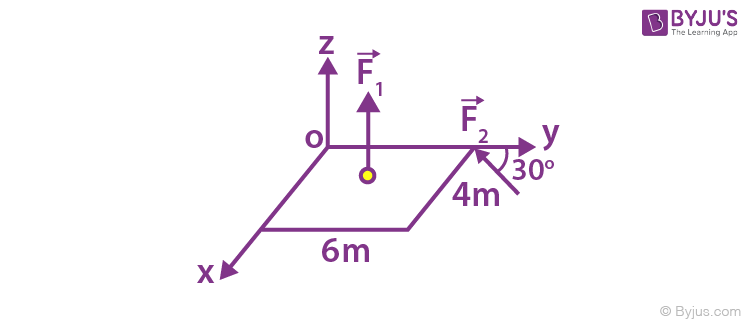a.

$$\begin{array}{l}(3\hat{i}-2\hat{j}-3\hat{k})F\end{array}$$

b.
$$\begin{array}{l}(3\hat{i}+2\hat{j}+3\hat{k})F\end{array}$$

c.
$$\begin{array}{l}(3\hat{i}+2\hat{j}-3\hat{k})F\end{array}$$

d.
$$\begin{array}{l}(3\hat{i}-2\hat{j}+3\hat{k})F\end{array}$$

Torque for F1 force

$$\begin{array}{l}\vec{F_{1}}=\frac{F}{2}(-\hat{i})+\frac{F\sqrt{3}}{2}(-\hat{j})\end{array}$$
$$\begin{array}{l}\vec{r_{1}}=0\hat{i}+6\hat{j}\end{array}$$
$$\begin{array}{l}\vec{\tau }_{F_{1}}=\vec{r _{1}}\times \vec{F _{1}}=3F\hat{k}\end{array}$$
$$\begin{array}{l}\vec{\tau }_{F_{2}}=\vec{r _{2}}\times \vec{F _{2}}=3F\hat{i}+2F(-\hat{j})\end{array}$$
$$\begin{array}{l}\vec{\tau }_{F_{net}}=\vec{\tau }_{F_{1}}+\vec{\tau }_{F_{2}}\end{array}$$
$$\begin{array}{l}=3F\hat{k}+3F\hat{i}+2F(-\hat{j})\end{array}$$

7. An equilateral triangle ABC is cut from a thin solid sheet of wood. (see figure) D, E and F are the mid-points of its sides as shown and G is the centre of the triangle. The moment of inertia of the triangle about an axis passing through G and perpendicular to the plane of the triangle is I0. It the smaller triangle DEF is removed from ABC, the moment of inertia of the remaining figure about the same axis is I. Then: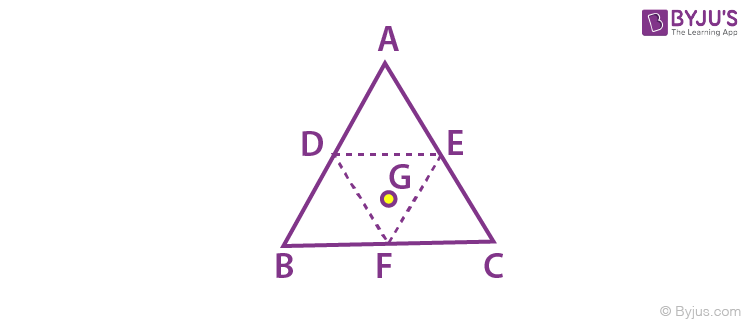a.

$$\begin{array}{l}I=\frac{9}{16}I_{0}\end{array}$$

b.
$$\begin{array}{l}I=\frac{3}{4}I_{0}\end{array}$$

c.
$$\begin{array}{l}I=\frac{1}{4}I_{0}\end{array}$$

d.
$$\begin{array}{l}I=\frac{15}{4}I_{0}\end{array}$$

Suppose M is mass and a is side of large triangle, then M/4 and a/2 will be mass and side length of smaller triangle.

$$\begin{array}{l}\frac{I_{removed}}{I_{original}}=\frac{\frac{M}{4}(\frac{a}{2})^{2}}{M(a)^{2}}\end{array}$$
$$\begin{array}{l}I_{removed}=\frac{I_{0}}{16}\end{array}$$
$$\begin{array}{l}I=I_{0}-\frac{I_{0}}{16}=\frac{15I_{0}}{16}\end{array}$$

8. A satellite is revolving in a circular orbit at a height h from the earth surface, such that h << R where R is the radius of the earth. Assuming that the effect of earth’s atmosphere can be neglected the minimum increase in the speed required so that the satellite could escape from the gravitational field of earth is :

a.

$$\begin{array}{l}\sqrt{gR}\end{array}$$

b.
$$\begin{array}{l}\sqrt{2gR}\end{array}$$

c.
$$\begin{array}{l}\sqrt{gR}(\sqrt{2}-1)\end{array}$$

d.
$$\begin{array}{l}\sqrt{\frac{gR}{2}}\end{array}$$

$$\begin{array}{l}v_{0}=\sqrt{g(R+h)}\approx \sqrt{gR}\end{array}$$
$$\begin{array}{l}v_{e}=\sqrt{2g(R+h)}\approx \sqrt{2gR}\end{array}$$
$$\begin{array}{l}\Delta v=v_{e}-v_{0}=(\sqrt{2}-1) \sqrt{gR}\end{array}$$

9. Ice at –20° C is added to 50 g of water at 40°C.When the temperature of the mixture reaches 0°C, it is found that 20 g of ice is still not melted. The amount of ice added to the water was close to

(Specific heat of water = 4.2 J/g/°C)

Specific heat of Ice = 2.1 J/g/°C

Heat of fusion of water at 0°C = 334 J/g)

a. 50 g
b. 40 g
c. 60 g
d. 100 g

Let amount of ice is m gm.

According to principal of calorimeter

heat taken by ice = heat given by water

Therefore, 20 × 2.1 × m + (m – 20) × 334

= 50 × 4.2 × 40

376 m = 8400 + 6680

m = 40.1 g

10. A rigid diatomic ideal gas undergoes an adiabatic process at room temperature. The relation between temperature and volume of this process is TVX= constant, then x is:

a. (5/3)
b. (2/5)
c. (2/3)
d. (3/5)

Diatomic gas

r=1 + (2/5) = 7/5

$$\begin{array}{l}PV^{r}= constant\end{array}$$
$$\begin{array}{l}(\frac{nRT}{V})V^{r}= C\end{array}$$
$$\begin{array}{l}TV^{r-1}=constant\end{array}$$
$$\begin{array}{l}TV^{r-1}=TV^x\end{array}$$

x=r-1 = (7/5) -1 = 2/5

11. A gas mixture consists of 3 moles of oxygen and 5 moles of argon at temperature T. Considering only translational and rotational modes, the total internal energy of the system is

a. 4RT
b. 12 RT
c. 15 RT
d. 20 RT

$$\begin{array}{l}f_{min}=\frac{n_{1}f_{1}+n_{2}f_{2}}{n_{1}+n_{2}}=\frac{(3\times 5)+(5\times 3)}{8}=\frac{15}{4}\end{array}$$
$$\begin{array}{l}U = \frac{f}{2}nRT= \frac{15}{8}\times 8\times RT = 15RT\end{array}$$

12. A particle undergoing simple harmonic motion has time dependent displacement given by

$$\begin{array}{l}x(t)=Asin\frac{\pi t}{90}\end{array}$$
.The ratio of kinetic to potential energy of this particle at t = 210 s will be

a. 1
b. 3
c. 1/9
d. 2

$$\begin{array}{l}k=\frac{1}{2}m\omega ^{2}A^{2}cos^{2}\omega t\end{array}$$
$$\begin{array}{l}U=\frac{1}{2}m\omega ^{2}A^{2}sin^{2}\omega t\end{array}$$
$$\begin{array}{l}\frac{k}{U}=cot^{2}\omega t=cot^{2}\frac{\pi }{90}(210)=1/3\end{array}$$

Hence ratio is 3

13. Equation of travelling wave on a stretched string of linear density 5 g/m is y = 0.03 sin (450 t – 9x) where distance and time are measured is SI units. The tension in the string is:

a. 12.5 N
b. 7.5 N
c. 10 N
d. 5 N

y = 0.03 sin(450 t – 9x)

$$\begin{array}{l}v=\frac{\omega }{k}=\frac{450}{9}=50\end{array}$$
m/s

$$\begin{array}{l}v=\sqrt{\frac{T}{\mu }}\Rightarrow \frac{T}{\mu }=2500\end{array}$$
$$\begin{array}{l}T = 2500\times 5\times 10^{-3}\end{array}$$

T = 12.5 N

14. In the figure shown below, the charge on the left plate of the

$$\begin{array}{l}10\mu F\end{array}$$
capacitor is
$$\begin{array}{l}-30\mu C\end{array}$$
. The charge on the right plate of the 6
$$\begin{array}{l}6\mu F\end{array}$$
capacitor is :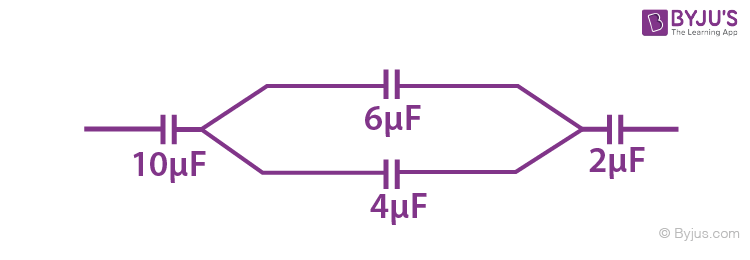a.

$$\begin{array}{l}-18\mu C\end{array}$$

b.
$$\begin{array}{l}-12\mu C\end{array}$$

c.
$$\begin{array}{l}+12\mu C\end{array}$$

d.
$$\begin{array}{l}+18\mu C\end{array}$$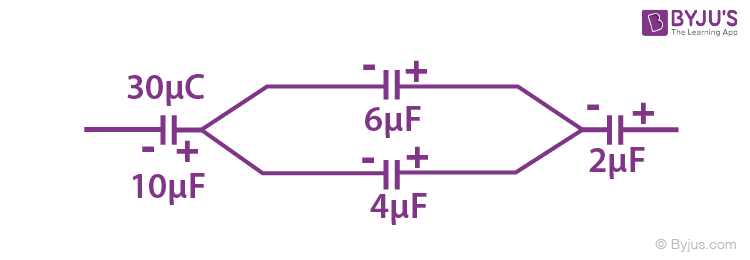6μF & 4μF are in parallel & total charge on this combination is 30 μC

Charge on 6μF capacitor = [6/(6+4)] x 30 = 18 μC

Since charge is asked on right plate therefore is +18μC

15. Three charges Q +q and +q are placed at the vertices of a right-angle isosceles triangle as shown below. The net electrostatic energy of the configuration is zero, if the value of Q is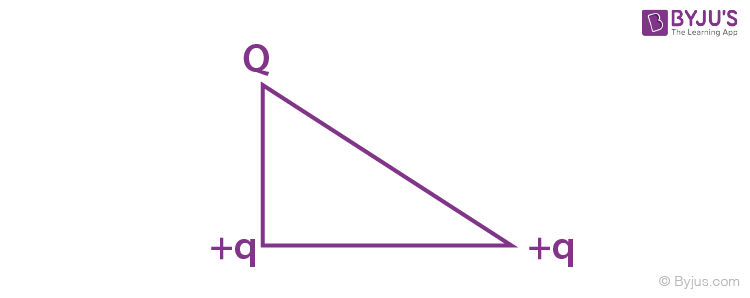a.

$$\begin{array}{l}-q/(1+\sqrt{2})\end{array}$$

b.
$$\begin{array}{l}-2q/(2+\sqrt{2})\end{array}$$

c. -2q
d. +q

Net electrostatic energy of the configuration will be

$$\begin{array}{l}U = K\left [ \frac{q.q}{a}+\frac{Q.q}{\sqrt{2}a} + \frac{Q.q}{a}\right ]\end{array}$$

Here,

$$\begin{array}{l}K=\frac{1}{4\pi \varepsilon _{0}}\end{array}$$

Putting ,U =0 we get,

$$\begin{array}{l}Q=\frac{-2q}{2+\sqrt{2}}\end{array}$$

16: The given graph shows variation (with distance r from centre) of: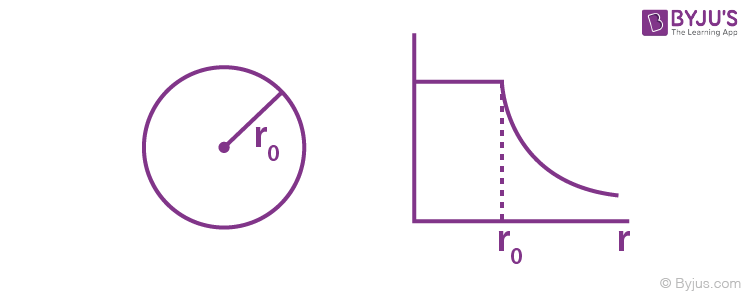a. Potential of a uniformly charged sphere
b. Potential of a uniformly charged spherical shell
c. Electric field of uniformly charged spherical shell
d. Electric field of uniformly charged sphere

Theory

$$\begin{array}{l}V=\frac{KQ}{R};r<R\end{array}$$
$$\begin{array}{l}V=\frac{KQ}{r};r>R\end{array}$$

17. Two equal resistance when connected in series to a batter, consume electric power of 60 W. If these resistances are now connected in parallel combination to the same battery, the electric power consumed will be:

a. 60 W
b. 240 W
c. 30 W
d. 120 W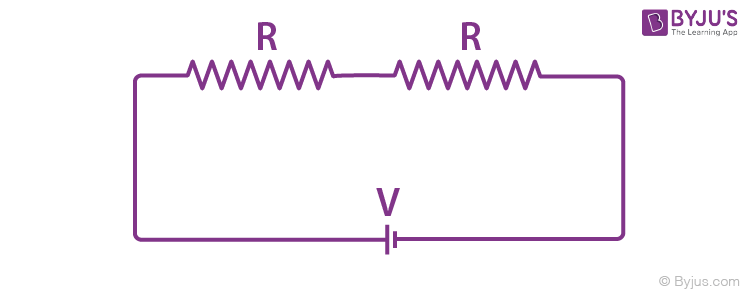In series condition, equivalent resistance is 2R

thus power consumed is 60W

P =60 = V2/2R

V2/R = 120 Watt

In parallel condition, equivalent resistance is R/2 thus new power isP = V2/(R/2) = 2(120)= 240W

18. In a Wheatstone bridge (see fig.), Resistances P and Q are approximately equal. When R = 400 Ω, the bridge is equal. When R = 400 Ω, the bridge is balanced. On inter-changing P and Q, the value of R, for balance, is 405 Ω. The value of X is close to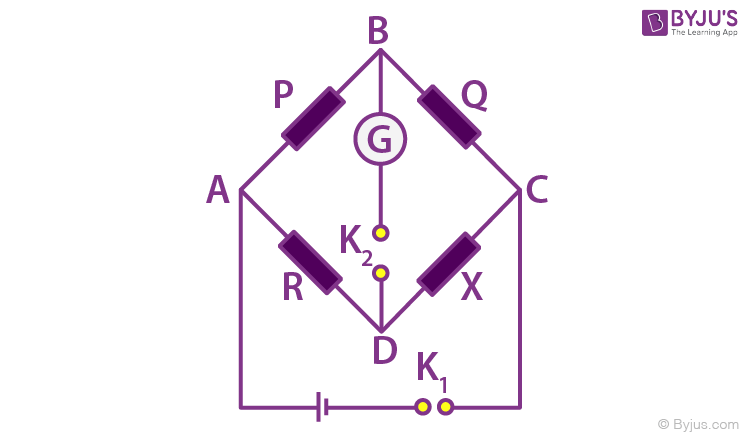a. 403.5 ohm
b. 404.5 ohm
c. 401.5 ohm
d. 402.5 ohm

Initially

$$\begin{array}{l}\frac{P}{Q}=\frac{R_{1}}{X}\end{array}$$
———–(1)

After interchanging P and Q

$$\begin{array}{l}\frac{Q}{P}=\frac{R_{2}}{X}\end{array}$$
———–(2)

From (i) and (ii) we have

$$\begin{array}{l}1= \frac{R_{1}R_{2}}{X^{2}}\end{array}$$

X =

$$\begin{array}{l}\sqrt{R_{1}R_{2}}=\sqrt{400\times 405}=402.5\Omega\end{array}$$

19. In an experiment, electrons are accelerated, from rest, by applying a voltage of 500 V.

Calculate the radius of the path if a magnetic field 100 mT is then applied.

[Charge of the electron = 1.6 × 10-19 C

Mass of the electron = 9.1 × 10–31 kg]

a. 7.5 × 10-4m
b. 7.5 × 10-3 m
c. 7.5 m
d. 7.5 × 10-2 m

$$\begin{array}{l}r=\frac{\sqrt{2mk}}{eB}=\frac{\sqrt{2me\Delta v}}{eB}\end{array}$$
$$\begin{array}{l}r=\frac{\sqrt{\frac{2m}{e}\Delta v}}{B}\end{array}$$
$$\begin{array}{l}r=\frac{\sqrt{\frac{2\times 9.1\times 10^{-31}}{0.16\times 10^{-19}}500}}{100\times 10^{-3}}\end{array}$$

r = 7.5 x 10-4 m

20. There are two long co-axial solenoids of same length l. the inner and outer coils have radii r1 and r2 and number of turns per unit length n1 and n2 respectively. The ratio of mutual inductance to the self-inductance of the inner-coil is:

a.

$$\begin{array}{l}\frac{n_{2}}{n_{1}}\frac{r_{2}^{2}}{r_{1}^{2}}\end{array}$$

b.
$$\begin{array}{l}\frac{n_{2}}{n_{1}}\frac{r_{1}}{r_{2}}\end{array}$$

c.
$$\begin{array}{l}\frac{n_{1}}{n_{2}}\end{array}$$

d.
$$\begin{array}{l}\frac{n_{2}}{n_{1}}\end{array}$$

Mutual Inductance=

$$\begin{array}{l} = \mu _{0}n_{1}n_{2}R_{2}^{2}l\end{array}$$

Self Inductance =

$$\begin{array}{l}= \mu _{0}n_{1}^{2}R_{2}^{2}l\end{array}$$

Ratio = n2/n1

21. In the circuit shown,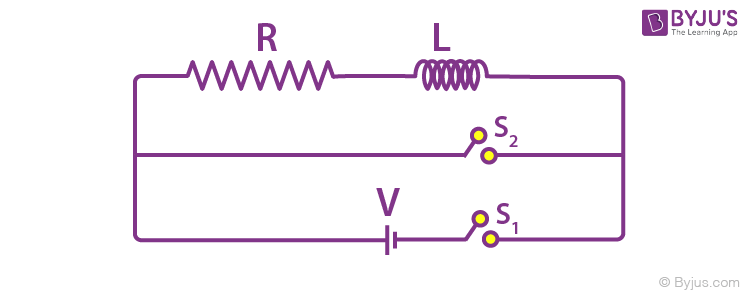the switch S1 is closed at time t = 0 and the switch S2 is kept open. At some later time (t0), the switch S1 is opened and S2 is closed. The behavior of the current I as a function of time ‘t’ is given by: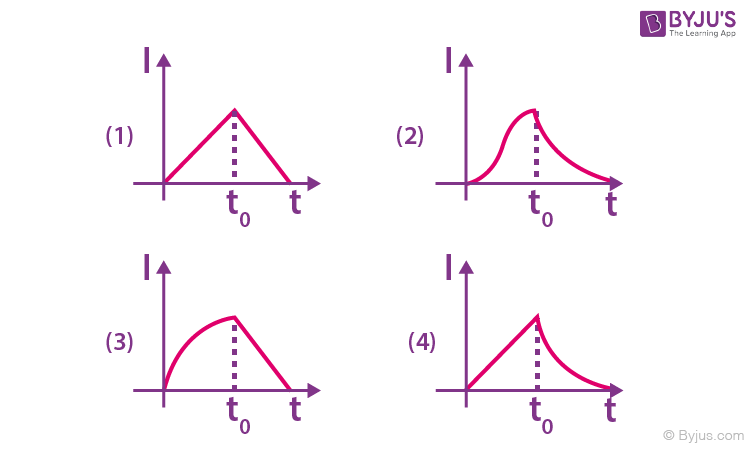From time t = 0 to t = t0, growth of current takes place and after that decay of current takes place.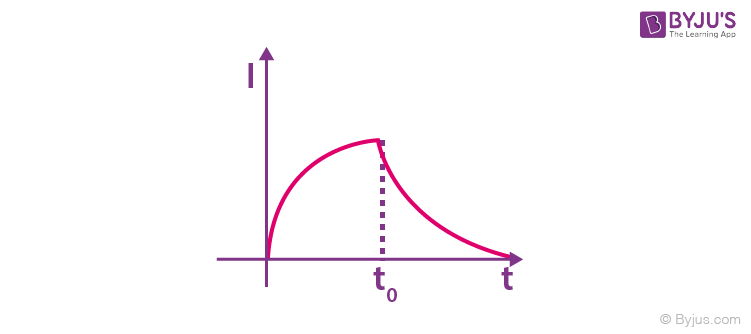22. An electromagnetic wave of intensity 50 Wm-2 enters in a medium of refractive index ‘n’ without any loss. The ratio of the magnitudes of electric fields, and the ratio of the magnitudes of magnetic fields of the wave before and after entering into the medium are respectively, given by:

a.

$$\begin{array}{l}\left [ \frac{1}{\sqrt{n}},\frac{1}{\sqrt{n}} \right ]\end{array}$$

b.
$$\begin{array}{l}\left [ \sqrt{n},\frac{1}{\sqrt{n}} \right ]\end{array}$$

c.
$$\begin{array}{l}\left [ \sqrt{n},\sqrt{n} \right ]\end{array}$$

d.
$$\begin{array}{l}\left [ \frac{1}{\sqrt{n}},\sqrt{n} \right ]\end{array}$$

$$\begin{array}{l}C=\frac{1}{\sqrt{\mu _{0}\epsilon _{0}}}\end{array}$$
$$\begin{array}{l}V=\frac{1}{\sqrt{k\mu _{0}\epsilon _{0}}}\end{array}$$

(For transparent medium

$$\begin{array}{l}\mu _{r}\approx \mu _{0}\end{array}$$
)

Therefore,

$$\begin{array}{l}\frac{C}{V}=\sqrt{k}=n\end{array}$$
$$\begin{array}{l}\frac{1}{2}\epsilon _{0}E_{0}^{2}C=intensity=\frac{1}{2} \epsilon _{0}kE^{2}y\end{array}$$
$$\begin{array}{l}E_{0}^{2}C=kE^{2}v\end{array}$$
$$\begin{array}{l}\Rightarrow \frac{E_{0}^{2}}{E^{2}}=\frac{kV}{C}=\frac{n^{2}}{n}\end{array}$$
$$\begin{array}{l}\Rightarrow \frac{E_{0}}{E}=\sqrt{n}\end{array}$$

Similarly,

$$\begin{array}{l}\frac{B_{0}^{2}C}{2\mu _{0}}=\frac{B^{2}C}{2\mu _{0}}\Rightarrow \frac{B_{0}}{B}=\frac{1}{\sqrt{n}}\end{array}$$

23. An object at a distance of 20m from a convex lens of focal length 0.3m. The lens forms an image of the object. If the object moves away from the lens at a speed of 5m/s, the speed and direction of the image will be

a. 3.22 × 10–3 m/s towards the lens
b. 2.26 × 10–3 m/s away from the lens
c. 1.16 × 10–3 m/s towards the lens
d. 0.92 × 10–3 m/s away from the lens

$$\begin{array}{l}\frac{dV}{dt}=\left (\frac{f}{f+u} \right )^{2}\frac{du}{dt}\end{array}$$

=

$$\begin{array}{l}\frac{dV}{dt}=\left (\frac{0.3}{0.3-20} \right )^{2}\times 5\end{array}$$

= 1.16 x 10-3 m/s towards lens

24. In a Young’s double slit experiment, the path difference, at a certain point on the screen between two interfering waves is (1/8)th of wavelength. The ratio of the intensity at this point to that at the centre of a bright fringe is close to

a. 0.74
b. 0.80
c. 0.85
d. 0.94

$$\begin{array}{l}I=I_{0}cos^{2}(\phi /2)= I_{0}cos^{2}\left ( \frac{\pi }{8} \right )\end{array}$$
$$\begin{array}{l}= I_{0}\left ( \frac{1+cos(\frac{\pi }{4})}{2} \right )\end{array}$$
$$\begin{array}{l}= I_{0}\left ( \frac{1+(\frac{1 }{\sqrt{2}})}{2} \right )\end{array}$$
$$\begin{array}{l}= (0.85)I_{0}\end{array}$$

25. If the deBroglie wavelength of an electron is equal to 103 times the wavelength of a photon of frequency 6×10 14 Hz, then the speed of electron is equal to : (Speed of light = 3×10 8m/s, Planck’s constant = 6.63×1034J . Mass of electron = 9.11031kg)

a. 1.8 x 106 m/s
b. 1.45 x 106 m/s
c. 1.1 x 106 m/s
d. 1.7 x 106 m/s

$$\begin{array}{l}\frac{h}{mV}=10^{-3}\left ( \frac{3\times 10^{8}}{6\times 10^{14}} \right )\end{array}$$
$$\begin{array}{l}V=\frac{6.63\times 10^{-34}\times 6\times 10^{14}}{9.1\times 10^{-31}\times 3\times 10^{5}}\end{array}$$

V = 1.45 x 106 m/s

26. A hydrogen atom, initially in the ground state is excited by absorbing a photon of wavelength 980Å. The radius of the atom in the excited state, in terms of Bohr radius a0, will be: (hc = 12500 eV – Å)

a. 9a0
b. 25a0
c. 4a0
d. 16a0

Energy of photon =12500/980 = 12.75eV

Electron will excite to n= 4

Since, R ∝ n2

Radius of atom will be 16a0

27. In the given circuit the current through Zener Diode is close to: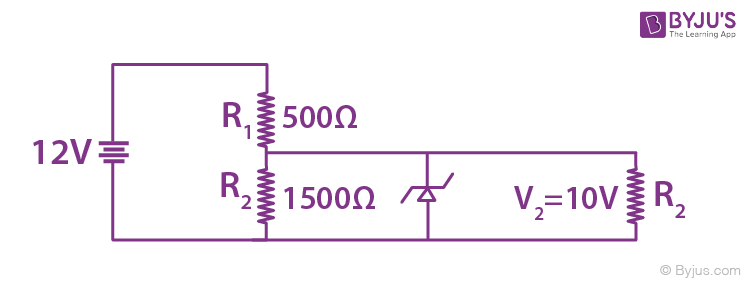a. 6.7 mA
b. 4.0 mA
c. 0.0 mA
d. 6.0 mA

Since voltage across zener diode must be less than 10V therefore it will not work in breakdown region, & its resistance will be infinite & current through it = 0

28. An amplitude modulated signal is given by V(t) = 10[1 + 0.3cos(2.2 × 104)]sin(5.5 × 105t). Here t is in seconds. The sideband frequencies (in kHz) are, [Given π = 22/7]

a. 1785 and 1715
b. 892.5 and 857.5
c. 89.25 and 85.75
d. 178.5 and 171.5

$$\begin{array}{l}V(t)=10+\frac{3}{2}\left [ 2cosAsinB \right ]\end{array}$$
$$\begin{array}{l}V(t)=10+\frac{3}{2}\left [ sin(A+B)-sin(A-B) \right ]\end{array}$$
$$\begin{array}{l}V(t)=10+\frac{3}{2}\left [ sin(57.2\times 10^{4}t)-sin(52.8\times 10^{4}t) \right ]\end{array}$$
$$\begin{array}{l}\omega _{1}=57.2\times 10^{4}=2\pi f_{1}\end{array}$$
$$\begin{array}{l}f_{1}=\frac{57.2\times 10^{4}}{2\times (\frac{22}{7})}=91KHz\end{array}$$
$$\begin{array}{l}f_{2}=\frac{52.8\times 10^{4}}{2\times (\frac{22}{7})}=84KHz\end{array}$$

29. The variation of refractive index of a crown glass thin prism with wavelength of the incident light is shown. Which of the following graphs is the correct one, if Dm is the angle of minimum deviation?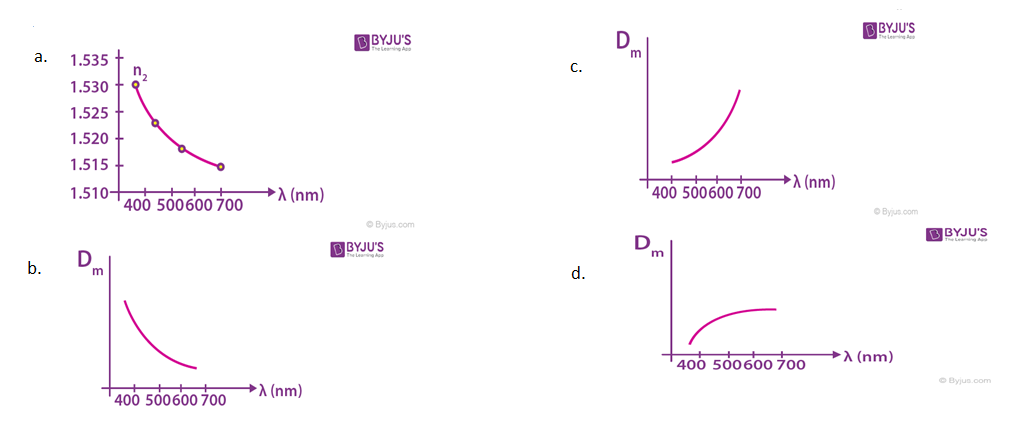Since Dm = (μ – 1)A and on increasing the wavelength, μ decreases and hence Dm decreases. Therefore, correct answer is (a)

30. The resistance of the meter bridge AB in the given figure is

$$\begin{array}{l}4\Omega\end{array}$$
. With a cell of emf = 0.5 V and rheostat resistance Rh =
$$\begin{array}{l}2\Omega\end{array}$$
the null point is obtained at some point J. When the cell is replaced by another one of emf
$$\begin{array}{l}\varepsilon =\varepsilon _{2}\end{array}$$
the same null point J is found for
$$\begin{array}{l}R_{h}=6\Omega\end{array}$$
. The emf
$$\begin{array}{l}\varepsilon _{2}\end{array}$$
is;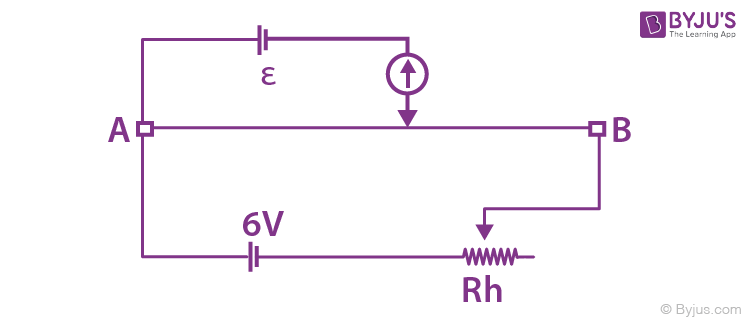a. 0.3 V
b. 0.5 V
c. 0.4 V
d. 0.6 V

Rh =

$$\begin{array}{l}2\Omega\end{array}$$
$$\begin{array}{l}i_{1}=\frac{6}{4+2}=1A\end{array}$$
$$\begin{array}{l}\varepsilon =\frac{1\times 4}{AB}\times AJ\end{array}$$
$$\begin{array}{l}AJ=\frac{AB}{4}\times \varepsilon\end{array}$$
———(1)

When Rh =

$$\begin{array}{l}6\Omega\end{array}$$
$$\begin{array}{l}i_{1}=\frac{6}{4+6}=0.6A\end{array}$$
$$\begin{array}{l}\varepsilon_{2} =\frac{0.6\times 4}{AB}\times AJ\end{array}$$
$$\begin{array}{l}AJ=\frac{AB\times \varepsilon _{2}}{0.6 \times 4}\end{array}$$
—-(2)

From (1) and (2)

$$\begin{array}{l}\frac{\varepsilon _{2}\times AB}{0.6\times 4}=\frac{\varepsilon \times AB}{4}\end{array}$$
$$\begin{array}{l}\varepsilon _{2}=0.6\times \varepsilon =0.6 \times 0.5 = 0.3V\end{array}$$